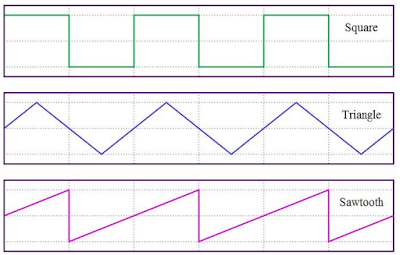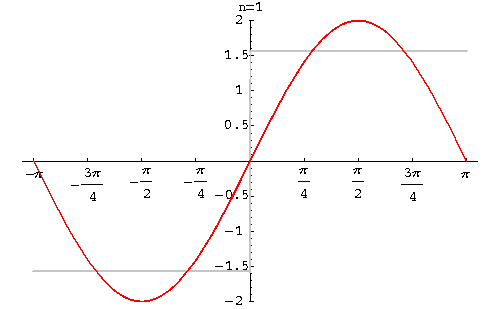## Pages

### Fourier Series

Fourier series is a way to represent a wavelike function as a sum of the 'sine' and 'cosine' functions. Some example of wavelike function are shown below,Photocourtesy: wikipedia

Definition: 'Let any function $f(x)$ be a $2\pi$-periodic function which is integrable in the interval $[-\pi,\pi]$ then the fourier series is given by,
$$f(x)=\frac{1}{2}a_{0}+\sum_{n=1}^{\infty }\left [ a_{n}cos(\frac{n\pi}{L}x)+b_{n}sin(\frac{n\pi}{L}x) \right ]$$
where,
$a_{0}=\frac{1}{\pi}\int_{-\pi}^{\pi}f(x)dx$,  $a_{n}=\frac{1}{\pi}\int_{-\pi}^{\pi}f(x)cos(\frac{n\pi}{L}x)dx$,  $b_{n}=\frac{1}{\pi}\int_{-\pi}^{\pi}f(x)sin(\frac{n\pi}{L}x)dx$

Details of the derivations of the fourier coefficient $a_{0}, a_{n}, b_{n}$ can be found here.

Exercise: Consider the following square-wave given below, Find the fourier expansion.

Sol$^{n}$:  Simple calculations shows that the fourier coefficient are,

$a_{0}=0$, $a_{n}=0$ and $b_{n}=\sum_{n=0}^{\infty}\frac{2}{2n+1}$

So, Substituting the coefficient in the fourier series, we have
$$f(x)=\sum_{0}^{\infty}\frac{2}{2n+1}sin((2n+1)x)$$

Expanding few terms up $n=6$ we have,
$$f(x)\approx2(sin(x)+\frac{1}{3}sin(3x)+\frac{1}{5}sin(5x)+\frac{1}{7}sin(7x)+\frac{1}{9}sin(9x)+\frac{1}{11}sin(11x)...)$$
The animation below justifies how the above sum approximate to the square waves,gifcourtesy:wikipedia

The animation below shows the further increasing the number of the terms to $n=21$,gifcourtesy:http://www.sosmath.com/

Here's an easy to understand video on introduction to fourier series,